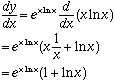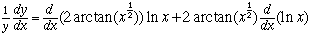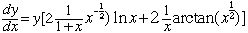Gina Renicker secondary asked by teacher The derivative of: y=e(xlnx) and y=x2arctan(x1/2) Hi Gina, To find the derivative of the first function you need to differentiate the exponential function and then apply the chain rule. If y=e(xlnx) thenThe key to the second problem is to take the natural log of both sides. Thus if y=x2arctan(x1/2) then lny = ln(x2arctan(x1/2)) = 2 arctan(x1/2) lnx Now differentiate both sides with respect to x to getand thusFinally replace y by y=x2arctan(x1/2) Cheers, Harley Go to Math Central